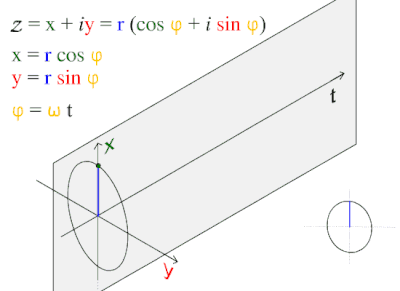# What does math.sin(tick()) mean?

This is a quick post, but I was simply wondering what math.sin(tick()) does in RunService.RenderStepped. I know that tick() is an amount of seconds since Jan 1st, 1970, why does the sine function return the incrementing/decrementing values from 1 to -1?

It makes it synchronous to time, and sine function is limited between -1 and 1 in output. If you know the equation for a sine wave on a graph, it’s simply:See how confined the values are, while `t` extends infinitely.

No matter how big the number is, the number will always return between -1 and 1.

6 Likes

just make a part, and set its Y position to math.sin(tick()) on renderstepped, you’ll understand it immediately
its just a wave

1 Like

So first of all, here is all about sine: ‘Sine - Wikipedia’ (I could write a book about it). `RunService.RenderStepped` runs prior to each frame being rendered on the client. A more descriptive version of your example would be:

``````local t = 0
local RunService = game:GetService('RunService')

RunService.RenderStepped:Connect(function(step)
-- Increment t with the time step
-- (time between last and current call of this event)
t += step
-- You can see how 't' evolves over time by 1 per second
print(math.sin(t), t)
end)
``````

I used step in combination with ‘t’ to show how `tick()` was used in your example.

1 Like